# Asset Correlation Matrix

## Definition

An Asset Correlation Matrix is a type of Correlation Matrix used in the context of a certain type of credit portfolio models

Asset correlation matrices can be estimated from the corresponding Equity Correlation Matrix but may incorporate additional transformations that take into account the liability structure of the firm. The underlying theory supporting the use of an asset matrix as input to estimating Default Correlation is that of Structural Credit Models.

While in principle asset correlations can be estimated directly between any two entities, in practice some factor structure may be employed:

## Sectoral / Regional Model

A common variation of the asset correlation matrix imposes as sectoral / regional structure

Under this factorization, if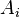$A_i$ is the asset process of an obligor,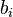$b_i$ its beta with a factor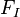$F_I$ and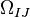$\Omega_{IJ}$ is the correlation between factors I and J, the asset correlation matrix is given by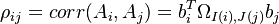$\rho_{ij} = corr(A_i, A_j) = b_i^T \Omega_{I(i),J(j)} b_j$